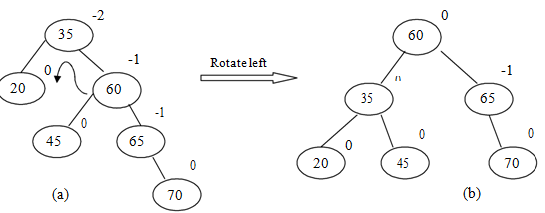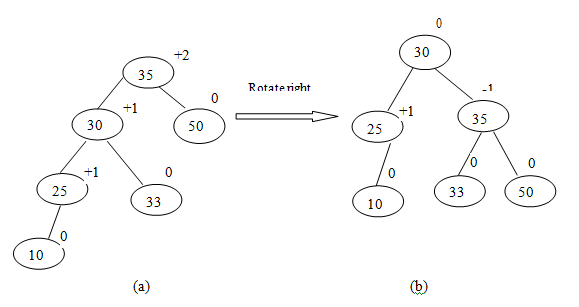## Rotations in binary tree, Data Structure & Algorithms

Assignment Help:

How can you rotate a Binary Tree? Explain right and left rotations by taking an example.

If after we have inserted a node in a Binary search tree, the balancing factor (height of left subtree - height of right subtree) of each node remains 0, 1 and -1 then there is no need of modification or alteration of original tree. If balancing factor of any node is either +2 or -2, the tree becomes unbalanced. It can be balanced by using left rotation or right rotation or a combination of both the rotations.

For example

1.  In the tree drawn below, after inserting node 70 , the balance factor of tree  at the root node (2) so the tree is rotated left to have a height balanced tree shown by (a) to (b):2.  In the tree drawn below, after inserting 10 the balance factor of tree at root node is +2 so the tree is rotated right to have height balanced tree as shown by(a) to (b) below:#### Implementation of queue, For a queue a physical analogy is a line at bookin...

For a queue a physical analogy is a line at booking counter. At booking counter, customers go to the rear (end) of the line & customers are attended to several services from the fr

#### Frequency count, i:=1 while(i { x:=x+1; i:=i+1; }

i:=1 while(i { x:=x+1; i:=i+1; }

#### Comp. sci algorithms, 1. develop an algorithm which reads two decimal numbe...

1. develop an algorithm which reads two decimal numbers x and y and determines and prints out wether x>y or y>x. the input values, x and y, are whole number > or equal to 0, which

#### What is diffuse illumination, Diffuse Illumination Diffuse illuminatio...

Diffuse Illumination Diffuse illumination means light that comes from all directions not from one particular source. Think about the light of a grey cloudy day as compared to

#### Red black tree, red black tree construction for 4,5,6,7,8,9

red black tree construction for 4,5,6,7,8,9

#### Define threaded binary tree, Threaded Binary Tree:- By changing the NUL...

Threaded Binary Tree:- By changing the NULL lines in a binary tree to special links known as threads, it is possible to perform traversal, insertion and deletion without using

#### Illustrate hls colour model, HLS Colour Model  This model has the doub...

HLS Colour Model  This model has the double-cone representation shown in Figure 3.40. The three colour parameters in this model are called hue (H), lightness (L), and Saturati

#### State the complex reallocation procedure, State the complex reallocation pr...

State the complex reallocation procedure Some languages provide arrays whose sizes are established at run-time and can change during execution. These dynamic arrays have an in

#### Functions for inserting and deleting at either end of deque, Q. Devise a re...

Q. Devise a representation for a given list where insertions and deletions can be made at both the ends. Such a structure is called Deque (which means Double ended queue). Write fu

#### Linear node is given by means of pointer, A linear collection of data eleme...

A linear collection of data elements where the linear node is given by means of pointer is known as Linked list